GCSE Maths Algebra

Sequences

# Sequences

Here we will learn about different types of sequences including arithmetic sequences, geometric sequences and quadratic sequences and how to generate them and find missing terms, along with special sequences like the fibonacci sequence. We will also learn how to find the nth term of linear sequence and the nth term of a geometric sequence and how to work out whether a particular number appears in a sequence.

There are also sequences worksheets based on Edexcel, AQA and OCR exam questions, along with further guidance on where to go next if you’re still stuck.

## What is a sequence?

A number sequence is a set of numbers that follow a particular pattern or rule to get  from term to term.

There are four main types of different sequences you need to know, they are arithmetic sequences, geometric sequences, quadratic sequences and special sequences.

### What is a sequence?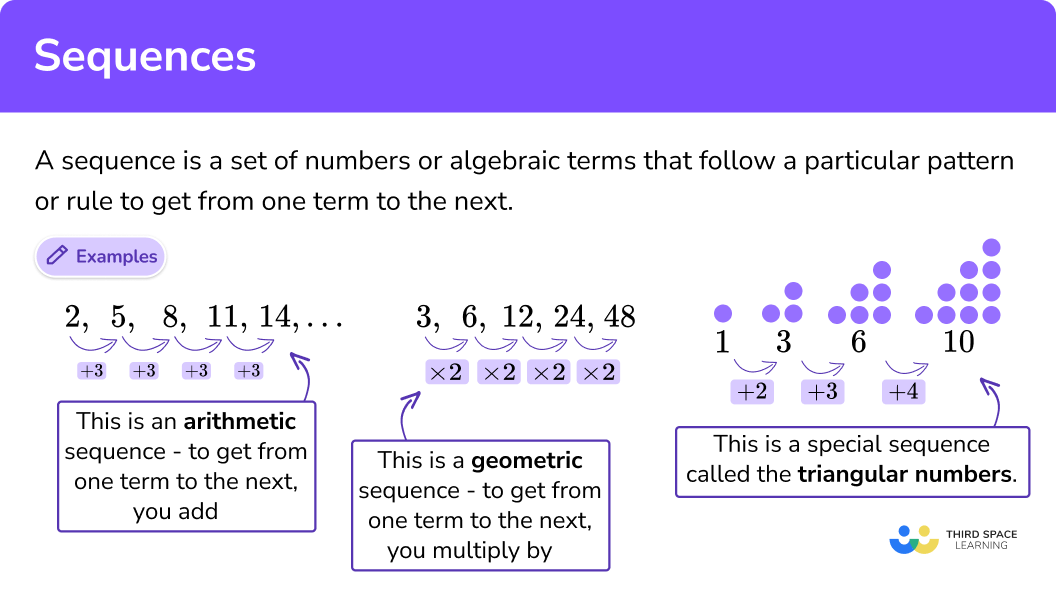1Arithmetic sequences

An arithmetic sequence is an ordered set of numbers that have a common difference between each term.

If we add or subtract by the same number each time to make the sequence, it is an arithmetic sequence

Step-by-step guide: Arithmetic Sequence

## Arithmetic sequences examples

### Example 1: sequence with a term to term rule of +3.

We add three to the first term to give the next term in the sequence, and then repeat this to generate the sequence.

### Example 2: sequence with a term to term rule of -1.

We subtract 1 from the first term to give the next term in the sequence, and then repeat this to generate the sequence.

We can work out previous terms by doing the opposite of the term to term rule.

2Geometric sequences

A geometric sequence is an ordered set of numbers that progresses by multiplying or dividing each term by a common ratio.

If we multiply or divide by the same number each time to make the sequence, it is a geometric sequence.

Step-by-step guide: Geometric sequences

## Geometric sequences examples

### Example 3: sequence with a term to term rule of ×2.

We multiply the first term by 2 to give the next term in the sequence, and then repeat this to generate the sequence.

### Example 4: sequence with a term to term rule of ÷2.

We divide the first term by 2 to give the next term in the sequence, and then repeat this to generate the sequence.

A quadratic sequence is an ordered set of numbers that follow a rule based on the sequence n2 = 1, 4, 9, 16, 25, … (the square numbers).

The difference between each term is not equal, but the second difference is.

### Example 5: sequence with common second difference of +2.

We find the first difference of the sequence and then find the term to term rule for the second difference. The second difference will always be the same for quadratic sequences.

4Special sequences

You will need to be able to recognise some important special sequences.

## Special sequences examples

### Example 6: square numbers

A square number is the result when a number is multiplied by itself.

E.g. 1×1=1, 2×2=4, 3×3=9 etc

The square numbers can form a sequence: 1, 4, 9, 16, 25, 36, 49…

nth Term = n2

### Example 7: cube numbers

A cube number is the result when a number is multiplied by itself three times.

E.g.

1×1×1=1, 2×2×2=8, 3×3×3=27 etc.

The cube numbers can form a sequence: 1, 8, 27, 64, 125

nth Term = n3

### Example 8: triangular numbers

The triangular numbers as numbers that can form a triangular dot pattern. They are also special type of quadratic sequence.

We can generate a sequence of triangular numbers by adding one more to the term to term rule each time:

### Example 9: Fibonacci numbers

We can generate the Fibonacci Sequence of numbers by adding the previous two numbers together to work out the next term.

First and second terms:

0+1=1, so the third term is 1.

Sequence: 0, 1, 1

Fourth Term:

1+1=2

Sequence: 0, 1, 1, 2

Fifth Term:

1+2=3

Sequence: 0, 1, 1, 2, 3

We can continue to follow the pattern to generate an infinite sequence.

The Fibonacci Sequence:

0, 1, 1, 2, 3, 5, 8, 13, 21, …

The Fibonacci Sequence forms a spiral that is seen throughout nature.

### What are the four main types of different sequences?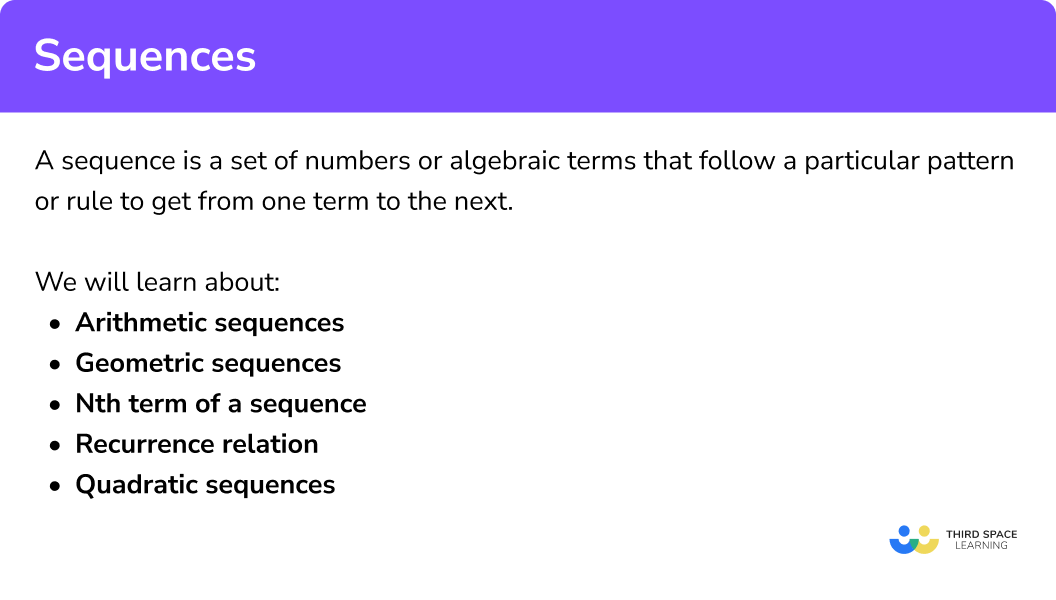## Sequence rule to find a term

We use the nth term of a sequence to work out a particular term in a sequence.
By substituting in the number of the term we want to find as ‘n’ we can generate the specific term in the sequence.

E.g.

What is the nth term and the 21st term of this sequence?

4, 10, 16, 22, …

The nth term of this sequence is 6n – 2.

To find the 21st term, n = 21

(6 x 21) – 2 = 124

The 21st term is 124.

E.g.

Given the nth term rule, 3n + 4, find the 20th and 100th term for this sequence.

To find the 20th term, n = 20

(3 x 20) + 4 = 64

To find the 100th term, n = 100

(3 x 100) + 4 = 304. The 20th term is 64 and the 100th term is 304.

## How to find the nth term of a sequence

The nth term is a formula that enables us to find any term in a sequence.

We will need to be able to find the nth term of a linear (arithmetic) sequence, and the nth term of a quadratic sequence

We can make a sequence using the nth term by substituting different values for the term number n into it.

Step-by-step guide: Nth term of a sequence

1nth term of a linear sequence

In order to find the nth term of a linear sequence:

• Step 1: find the common difference for the sequence.
• Step 2: multiply the values for n = 1, 2, 3, … by the common difference.
• Step 3: add or subtract a number to obtain the sequence given in the question.

### How to find the nth term of a linear sequence### nth term of a linear sequence example

Find the nth term for the sequence 5, 9, 13, 17, 21, ….

The common difference d = 4.

Here, we generate the sequence 4n=4, 8, 12, 16, 20, …. (the 4 times table).

The nth term of this sequence is 4n+1.

2nth term of a quadratic sequence

In order to find the nth term of a quadratic sequence we have to find the second difference. To do this, we calculate the first difference between each term and then calculate the difference between this new sequence.

• Step 1: find the first difference (d1) and second difference (d2) for the sequence.
• Step 2: Halve the second difference to find a, the coefficient of n2.

$\left(\frac{d_{2}}{2}=a\right)$
• Step 3: Subtract an2 from the original sequence.
• Step 4: If this produces a linear sequence, find the nth term of it.
• Step 5: Add the nth term for the linear sequence to an2 to work out the nth term of the quadratic sequence.

### How to find the nth term of a quadratic sequence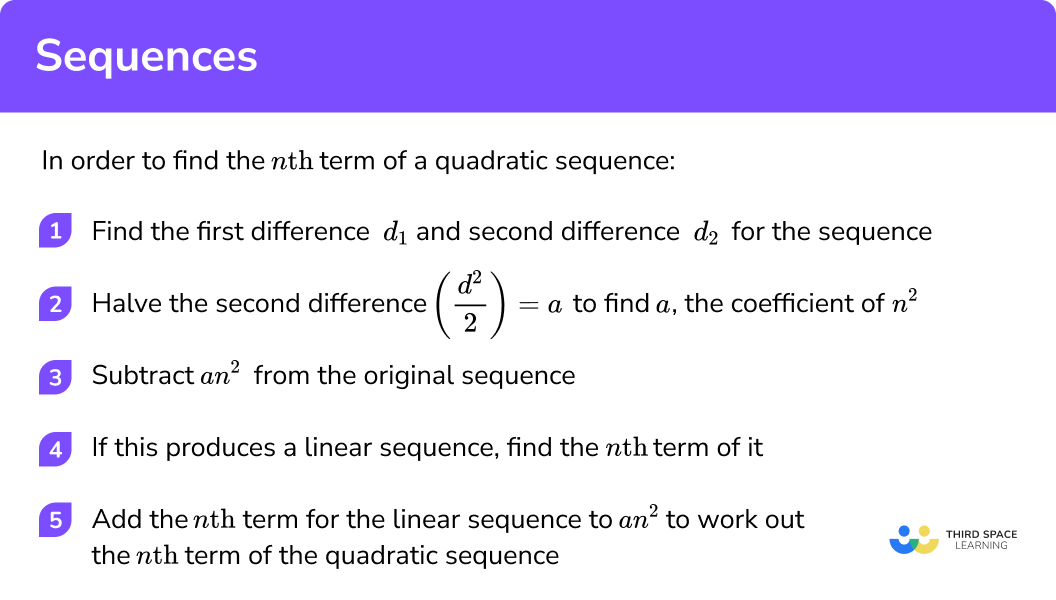### nth term of a quadratic sequence example

Find the nth term rule of the quadratic sequence:

5, 14, 29, 50, 77, …

The second difference d2=6.

$\left(\frac{d_{2}}{2}=a\right)$

As d2 = 6,

d2 ÷ 2 = 6 ÷ 2 = 3

This means a = 3 and so we have the sequence 3n2.

Here, the remainder for each term is 2.

Not required for this example as the remainder is 2 for each term.

The nth term of the quadratic sequence is 3n2+2.

3Use the nth term to calculate any term in a sequence

We can calculate any term in a sequence by substituting the term number into the nth term.

### Example 10: find terms in the sequence with nth term = 4n+1

• For the first term, n=1

So 1st term = 41 + 1 = 5

• For the 10th term, n=10

So 10th term = 4 ✕ 10 + 1= 41

• For the 100th term, n=100

So 100th term = 4 ✕ 100 + 1 = 401

### Example 11: find the first three terms in the sequence n2 + 7n

• For the first three terms we use n=1, n=2 and n=3:
n=1

1^{2} + 7 × 1 = 8

n=2

2^{2} + 7 × 2 = 18

n=3

3^{2} + 7 × 3 = 30

4Use the nth term to work out whether a number is in a sequence

We can use the nth term to work out whether a number is in a sequence by putting the nth term equal to the number and solving the equation to find n.

Because n is the term number it has to be an integer (a whole number).

i.e. for the 1st term n=1, for the 9th term n=9 etc.

When n is an integer then the number is in the sequence.

## nth term to work out whether a number is in a sequence examples

### Example 12: when n is an integer then the number is in the sequence

Is 25 a number in the sequence generated by the nth term 4n+1?

Let’s put 25 equal to 4n+1 and solve the equation.

\begin{aligned} 4n+1&=25 \\ 4n&=24 \\ n&=6 \end{aligned}

As 6 is an integer this means that 25 is the 6th term in the sequence.

So 25 is a number in the sequence.

### Example 13: when n is not an integer then the number is not in the sequence

Is 60 a number in the sequence generated by the nth term 4n+1?

Let’s put 60 equal to 4n+1 and solve the equation.

\begin{aligned} 4n+1&=60 \\ 4n&=59 \\ n&=14.75 \end{aligned}

As 14.75 is not an integer this means that 60 is not a number in the sequence.

5Solve problems using algebra in sequences

We can write any type of sequence described above using algebraic terms rather than numerical terms.

For example, the sequence,

3a  ,   3a+2  ,   3a+4   ,  3a+6

is an arithmetic sequence, because we add 2 each time to get from one term to the next.

We can apply skills such as solving linear equations and solving simultaneous equations to sequences with algebraic terms.

### Example 14: a Fibonacci sequence

The first three terms of a Fibonacci sequence are,

a  ,   a+2  ,   2a+2

The 3rd term is 12.

Find the value of the 4th term.

First we form and solve an equation using the information given about the 3rd term:

2 a+2 =12

2 a =10

a =5

We also need to find an algebraic expression for the 4th term. To get the next term in a Fibonacci sequence, we add the two previous terms. So the 4th term will be the sum of the 2nd and 3rd terms:

(a+2)+(2a+2)=3a+4

To find the value of the 4th term, substitute a=5 to get 3(5)+4=19

So the 4th term is 19.

Step-by-step guide: Sequences algebra

### Common misconceptions

• Mixing up arithmetic and geometric and quadratic sequences
• Arithmetic sequences are generated by adding or subtracting the same amount each time – they have a common difference d.
• Geometric sequences are generated by multiplying or dividing by the same amount each time – they have a common ratio r.
• Quadratic sequences have a common second difference d2.
• Mixing up working out a term in a sequence with whether a number appears in a sequence

In order to find any term in a sequence using the nth term we substitute a value for the term number into it.

E.g.

4n+1

• For the 5th term n=5

So 5th term=4(5)+1=21

• For the 8th term n=8

So 8th term=4(8)+1=33

In order to work out whether a number appears in a sequence using the nth term we put the number equal to the nth term and solve it. If n (the term number) is an integer the number is in the sequence, if n is not an integer the number is not in the sequence.

E.g.

Is 22 a number in the sequence with nth term = 4n+1?

4n+1=22

4n=21

n=5.25

As 5.25 is not an integer this means that 22 is not a number in the sequence.

### Practice sequences questions

1. Find the nth term of 1, 5, 9 ,13, …

4n-3n+44n+14nThe common difference here is 4 so it is 4n .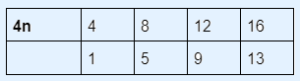To get from 4n to our sequence we need to subtract 3 so our sequence is 4n-3 .

2. Find the nth term of 7, 4, 1,-2,-5, …

7n-3n-3-3n+4-3n+10The common difference here is -3 so it is -3n .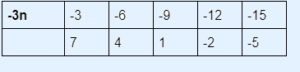To get from -3n to our sequence we need to add 10 so our sequence is -3n+10 .

3. Find the nth term of 6, 17, 32, 51, 74

n^{2}+52n^{2}+5n-111n-54n^{2}+2Here the second difference is 4 .
4 \div 2 = 2

So it is 2n^{2}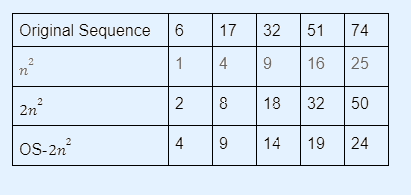We now need to work out the nth term formula for 4, 9, 14, 19, 24 ,..

The difference is 5 so it is 5n .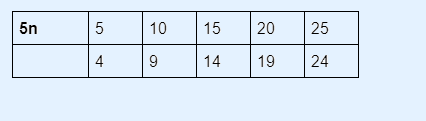To get from 5n to our sequence we need to subtract 1 so it is 5n-1 .

The overall formula for the sequence is 2n^{2}+5n-1.

4. Generate the first 6 terms of the sequence 3n-4

-4, -1, 2,5, 8, 113, -1, -5, -9, -13, -174, 12, 36, 108, 324, 972-1, 2, 5,8, 11, 14,…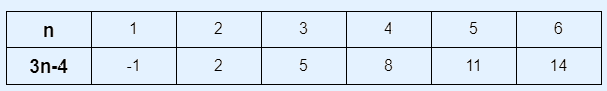5. Work out the 10 th term of the sequence 25-n^{2}

155-75-100n=10

\begin{aligned} 25-10^{2}&=25-100\\ &=-75\end{aligned}

6. Write down the next three terms in this sequence: 0, 1, 1, 2, 3, 5, 8, …

11, 14, 1713, 21, 3412, 17, 2314, 25, 47This is the Fibonacci Sequence. We add the two previous terms to get the next term:

### Sequences GCSE questions

1. (a) 5, 8, 11, 14

What is the rule to get from one term to the next?

(b) What is the nth term of the sequence?

(2 marks)

+3

(1)

3n+2

(1)

2.(a)What is the 12 th term in the sequence 2n-20 ?

(b) Is 180 a number in the sequence?

(3 marks)

4

(1)

##### \begin{aligned} 2n-20&=180\\ 2n&=200\\ n&=100 \end{aligned}

(1)

Yes because n is an integer. 180 is the 100 th term in the sequence.

(1)

3. Look at this sequence 1, 2, 4, 8, 16

(a) What is the common ratio in the above geometric sequence?

(b) What would be the 7 th and 8 th term for the sequence?

(3 marks)

##### 2

(1)

7 th term= 32 \times 2 = 64

(1)

8 th term= 64 \times 2 = 128

(1)

## Learning checklist

You have now learned how to:

• Recognise arithmetic sequences and find the nth term.
• Recognise and use sequences of triangular, square and cube numbers, simple arithmetic progressions, Fibonacci Sequences, quadratic sequences, and simple geometric progressions.
• Generate terms of a sequence from either a term-to-term or a position-to-term rule.
• Recognise and use quadratic sequences.
• Deduce expressions to calculate the nth term of quadratic sequences.

## Still stuck?

Prepare your KS4 students for maths GCSEs success with Third Space Learning. Weekly online one to one GCSE maths revision lessons delivered by expert maths tutors.

Find out more about our GCSE maths tuition programme.